WORKING STRESS

# 1 WORKING STRESS

## 1.1 Problem definition

Determinate the necessary cross section areas of the bars and deflections of the point B.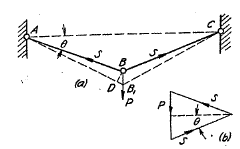Figure 1: Model by Timoshenko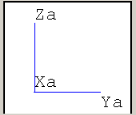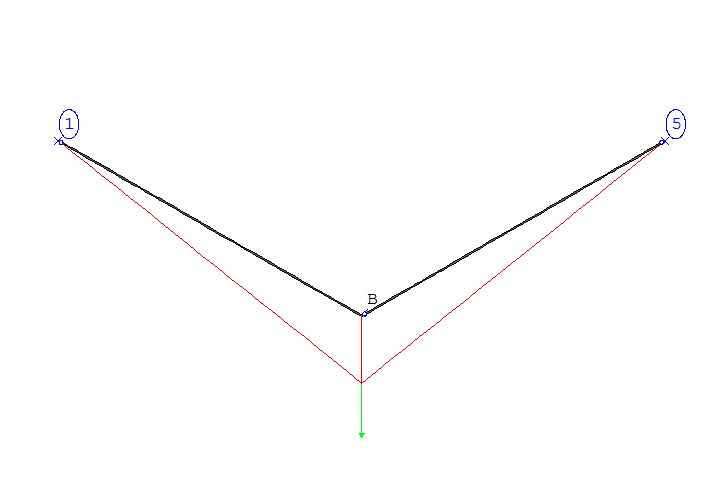Figure 2:ROHR2 model

## 1.2 References (Timoshenko)

S. Timoshenko, Strength of Material, Part I, Elementary Theory and Problems, 3rd Edition, D. Van Nostrand Co., Inc., New York, NY, 1956, Chapter 1.4, pg. 9-10.

A structure consisting of two equal carbon steel bars are submitted to a vertical force. The following parameters are given:

• Angle of inclination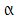• Modulus of Elasticity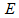• Length of both bars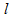• Stress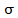• Force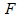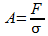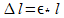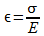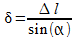Where:

 Variable Description Unit Used Value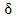Deflection inch 0,12Angle of inclination deg 30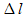Length variation inch 0,06Stress lbs/ inchÂ² 10000Modulus of Elasticity lbs/ inchÂ² 30000000Length of both bars inch 180Force lbf 5000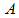Cross section inchÂ² 0,5

Table 1: Overview of the variables used

## 1.3 Model description (ROHR2)

The ROHR2 model consist two equal beams which are 180 inch long with a self-defined steel bar. The beam is made of carbon steel (cross section 0,5 inchÂ² â‰™ 3.2258 cmÂ²). At the middle a downward (-Z direction) vertical force (5000 lbf) is applied. Both bars are fixed at each end (Point 1,5). Additionally the points 1, B and 5 have the same boundary conditions (section moment MX isn't transmitted). As no acceleration due to gravity is to be considered, the line mass of the structural section is set to 0 lb/ ft.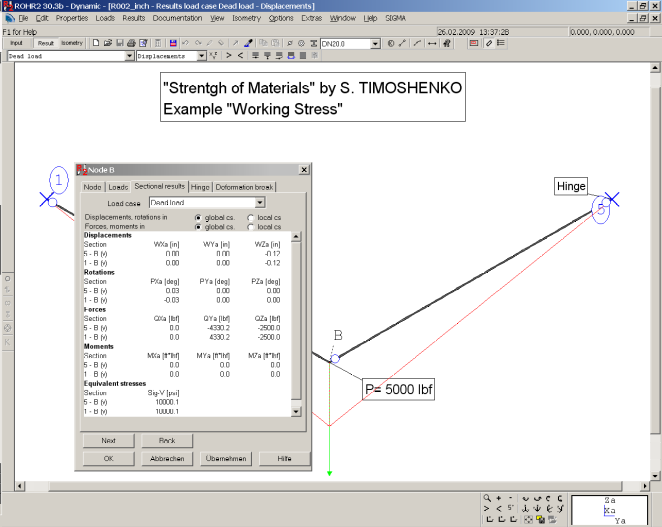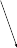Figure 3: Model with sectional results

## 1.4 Result comparisons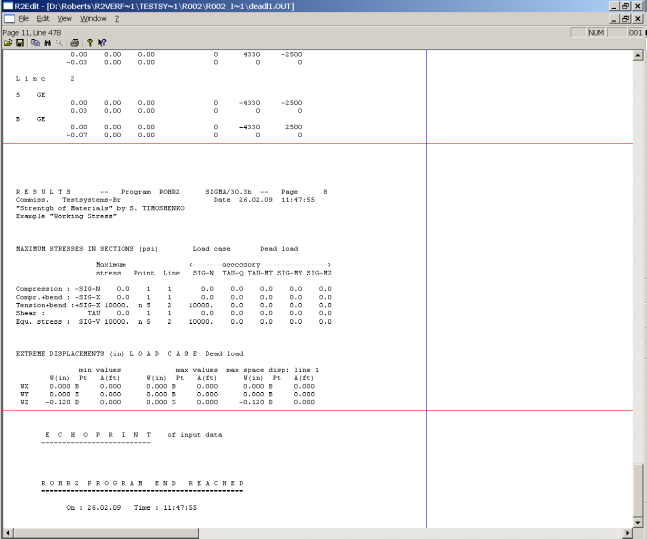Figure 4: Î´ from the Lc Dead Weight

 Value Length [inch] Reference (Timoshenko) [inch] Rohr2 [inch] Difference [%]180 0,012 0,012 <0,01

Table 2: Comparison of the deflection at point B

 Value Cross section (Timoshenko) [inchÂ²] Cross section (Rohr 2) [inchÂ²] Difference [%]0,5 0,5 <0,01

Table 3: Comparison of the cross section

## 1.5 Conclusion

The results are exact up to the limit of the given digits.

## 1.6 Files

R002_inch.r2w

R002_mm.r2w

R2_stresses_2.ods

SIGMA Ingenieurgesellschaft mbH www.rohr2.com